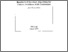# Regularized Gradient Algorithm for Convex Problems with Constraints

Aubin, J.-P. (1992). Regularized Gradient Algorithm for Convex Problems with Constraints. IIASA Working Paper. IIASA, Laxenburg, Austria: WP-92-066Preview Text WP-92-066.pdf Download (115kB) | Preview

## Abstract

Nesterov has proved the convergence of the discrete subgradient algorithm for minimizing convex finite functions bounded from below.

When the objective function is a lower semicontinuous convex extended function (which happens when one minimizes problems with constraints), the subgradient algorithm no longer makes sense since we do not know whether the iterations belong to the domain of the objective function.

Hence the idea is to approximate the objective function by its Moreau-Yosida approximation, which is differentiable, and to use the gradient algorithm applied to this approximation. We prove the convergence when both the steps of the algorithm converge to infinity and the Moreau-Yosida parameter converges to 0.

Item Type: Monograph (IIASA Working Paper) Adaption and Optimization (ADO) IIASA Import 15 Jan 2016 02:01 27 Aug 2021 17:14 https://pure.iiasa.ac.at/3633View Item